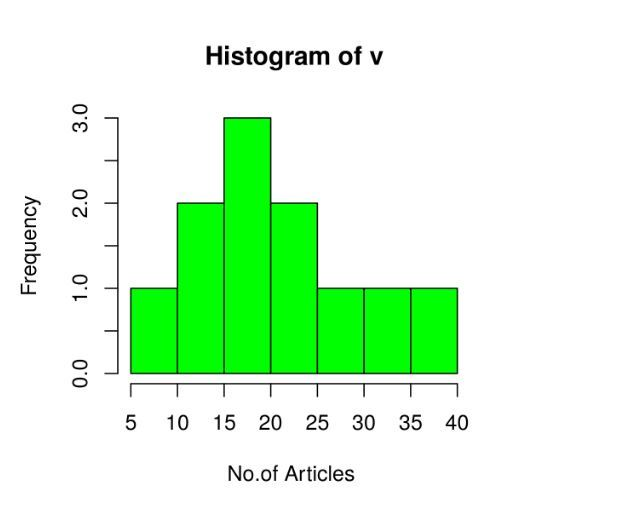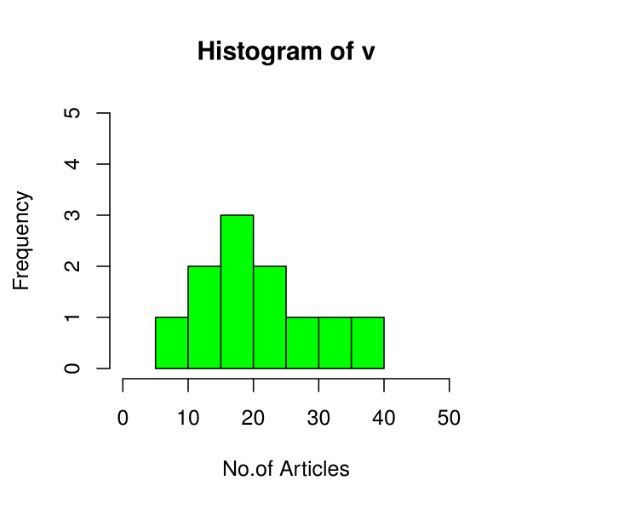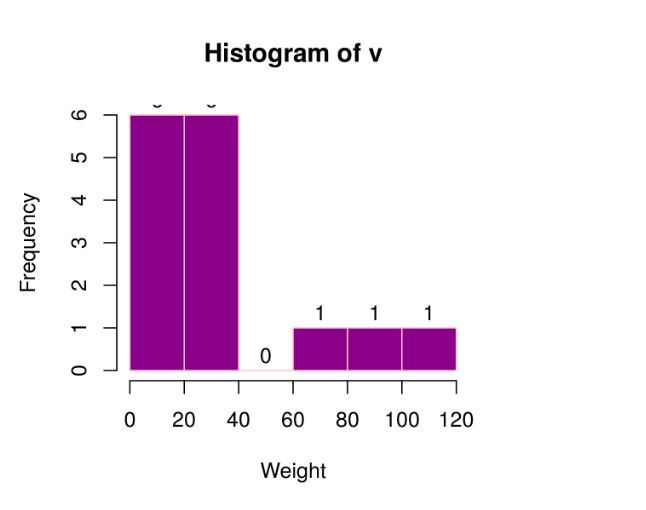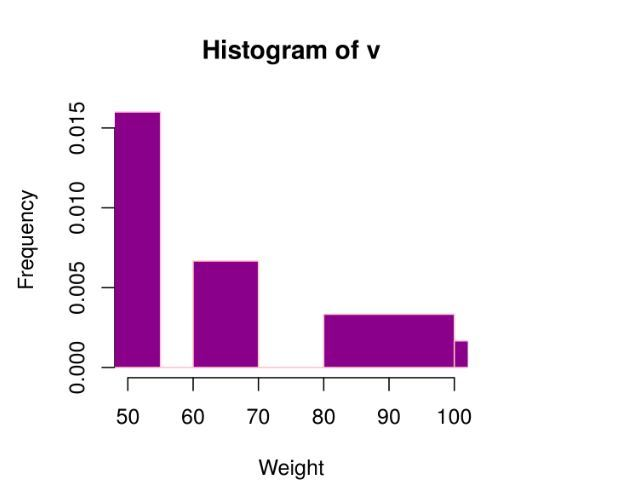# Histograms in R language

A histogram contains rectangular area to display the statistical information which is proportional to the frequency of a variable and its width in successive numerical intervals. A graphical representation that manages a group of data points into different specified ranges. It has a special feature which shows no gaps between the bars and similar to a vertical bar graph.

R creates histogram using hist() function.

Syntax:

`hist(v, main, xlab, xlim, ylim, breaks, col, border)`

Parameters:

• v: This parameter contains numerical values used in histogram.
• main: This parameter main is the title of the chart.
• col: This parameter is used to set color of the bars.
• xlab: This parameter is the label for horizontal axis.
• border: This parameter is used to set border color of each bar.
• xlim: This parameter is used for plotting values of x-axis.
• ylim: This parameter is used for plotting values of y-axis.
• breaks: This parameter is used as width of each bar.

### Creating a simple Histogram

Approach: In order to create Histogram chart:

1. Creating a simple histogram chart by using the above parameter.
2. This vector v is plot using hist().

Example:

 `# Create data for the graph. ` `v <``-`  `c(``19``, ``23``, ``11``, ``5``, ``16``, ``21``, ``32``, ``14``, ``19``, ``27``, ``39``) ` ` `  `# Create the histogram. ` `hist(v, xlab ``=` `"No.of Articles "``, col ``=` `"green"``, border ``=` `"black"``) `

Output:### Range of X and Y values

Approach: To describe the range of values we need to do following steps:

1. We can use the xlim and ylim parameter in X-axis and Y-axis.
2. Take all parameters which are required to make histogram chart.

Example

 `# Create data for the graph. ` `v <``-` `c(``19``, ``23``, ``11``, ``5``, ``16``, ``21``, ``32``, ``14``, ``19``, ``27``, ``39``) ` `  `  `  `  `# Create the histogram. ` `hist(v, xlab ``=` `"No.of Articles"``, col ``=` `"green"``,  ` `     ``border ``=` `"black"``, xlim ``=` `c(``0``, ``50``), ` `     ``ylim ``=` `c(``0``, ``5``), breaks ``=` `5``)      `

Output:### Using histogram return values for labels using text()

Approach: To create histogram return value chart:

 `# Creating data for the graph.   ` `v <``-`  `c(``19``, ``23``, ``11``, ``5``, ``16``, ``21``, ``32``, ``14``, ``19``, ``27``, ``39``, ``120``, ``40``, ``70``, ``90``)   ` ` `  `# Creating the histogram.   ` `m<``-``hist(v, xlab ``=` `"Weight"``, ylab ``=``"Frequency"``,  ` `        ``col ``=` `"darkmagenta"``, border ``=` `"pink"``, breaks ``=` `5``)   ` ` `  `# Setting labels   ` `text(m\$mids, m\$counts, labels ``=` `m\$counts, adj ``=` `c(``0.5``, ``-``0.5``))       `

Output: When we execute the above code, it shows the following result-### Histogram using non-uniform width

Approach: creating different width histogram chart:

1. By using above parameters, we created histogram using non-uniform width.

Example

 `# Creating data for the graph.   ` `v <``-`  `c(``19``, ``23``, ``11``, ``5``, ``16``, ``21``, ``32``, ``14``, ``19``, ``27``, ``39``, ``120``, ``40``, ``70``, ``90``)  ` `    `  `# Creating the histogram.   ` `hist(v, xlab ``=` `"Weight"``, ylab ``=``"Frequency"``, xlim ``=` `c(``50``, ``100``), ` `     ``col ``=` `"darkmagenta"``, border ``=` `"pink"``,  ` `     ``breaks ``=` `c(``5``, ``55``, ``60``, ``70``, ``75``, ``80``, ``100``, ``140``))    `

Output:My Personal Notes arrow_drop_upIf you like GeeksforGeeks and would like to contribute, you can also write an article using contribute.geeksforgeeks.org or mail your article to contribute@geeksforgeeks.org. See your article appearing on the GeeksforGeeks main page and help other Geeks.

Please Improve this article if you find anything incorrect by clicking on the "Improve Article" button below.

Article Tags :

Be the First to upvote.

Please write to us at contribute@geeksforgeeks.org to report any issue with the above content.